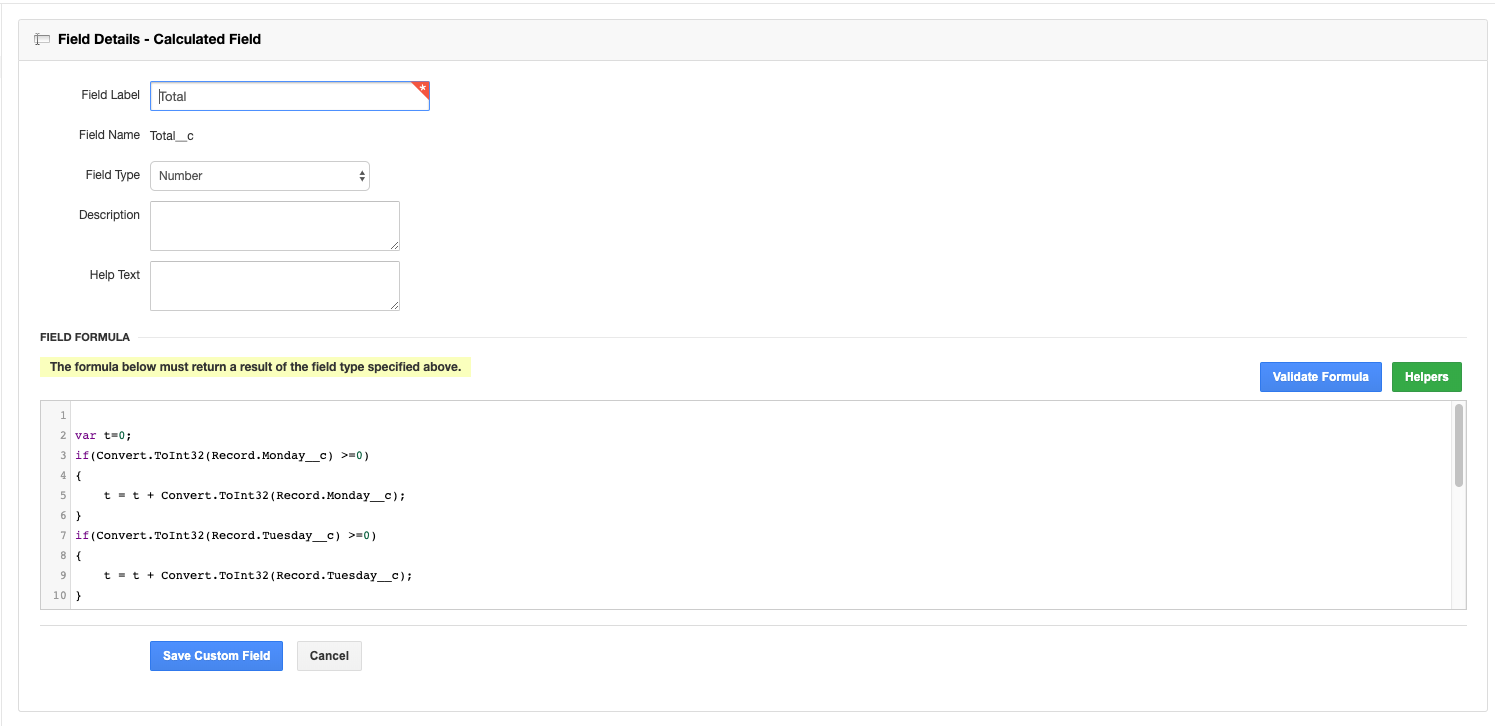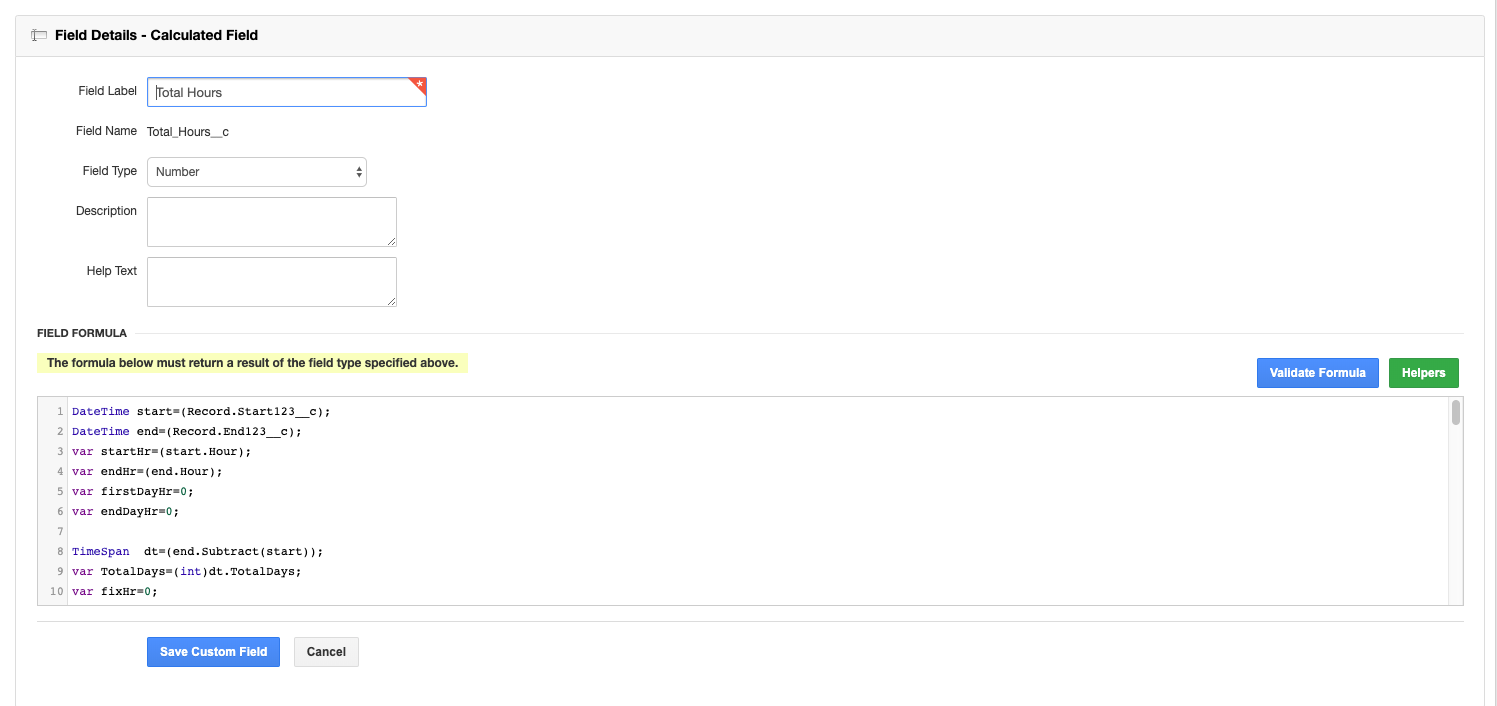# Custom Calculated Fields examples: Time Tracking with Tasks

Below are some examples of custom calculated fields and their use cases. This article focuses on the formulas that will help you implement time tracking.

With custom calculated fields, you can create mathematical formulas using C# (C sharp). The formulas and their resulting values are based on the data in your records.

We know that many of our users are not familiar with C# (C sharp) or any other C programming language. Don't worry - you can start using these formulas even if you have no coding knowledge.

Just copy and paste the formulas below into the Field Formula text box when you are creating a field.

Time Tracking examples

## Example #1: Calculate "Start Date" and "Due Date" differenceUse this formula to calculate the total number of days between the set Start Date and the set Due date.`DateTime start=(Record.START_DATE).ToUniversalTime();DateTime due=(Record.DUE_DATE).ToUniversalTime();TimeSpan dt=(due.Subtract(start));var TotalDays=(int)dt.TotalDays;return (int)dt.TotalDays; `

## Example #2: Total working hoursUse this formula to record hours for each day of the week and calculate the total number of hours overall.

You will need to create the following custom fields first:

• Monday
• Field Type = Numeric
• Label = Monday
• Tuesday
• Field Type = Numeric
• Label = Tuesday
• Wednesday
• Field Type = Numeric
• Label = Wednesday
• Thursday
• Field Type = Numeric
• Label = Thursday
• Friday
• Field Type = Numeric
• Label = Friday`var t=0;if(Convert.ToInt32(Record.Monday__c) >=0){    Total = Total + Convert.ToInt32(Record.Monday__c);}if(Convert.ToInt32(Record.Tuesday__c) >=0){    Total = Total + Convert.ToInt32(Record.Tuesday__c);}if(Convert.ToInt32(Record.Wednesday__c) >=0){    Total = Total + Convert.ToInt32(Record.Wednesday__c);}if(Convert.ToInt32(Record.Thursday__c) >=0){    Total = Total + Convert.ToInt32(Record.Thursday__c);}if(Convert.ToInt32(Record.Friday__c) >=0){    Total = Total + Convert.ToInt32(Record.Friday__c);}return t;`

## Example #3: Calculate hours between two datesUse this formula to calculate the number of hours between two dates, with a limit of 8 hours per day.

You will need to create the following custom fields first:

• Start Date
• Field Type = Date/Time
• Label = Start123
• End Date
• Field Type = Date/Time
• Label = End123`DateTime start=(Record.Start123__c);DateTime end=(Record.End123__c);var startHr=(start.Hour);var endHr=(end.Hour);var firstDayHr=0;var endDayHr=0;TimeSpan dt=(end.Subtract(start));var TotalDays=(int)dt.TotalDays;var fixHr=0;if(TotalDays==0){    var tot=(int)dt.TotalHours;    if(tot>8)    {         return 8;    }    else    {        return tot;    }}else{    if(TotalDays>1)    {          fixHr=(TotalDays - 1) *8;    }//Calculation for start day hours    if(startHr>=7 && startHr<=14)    {          startHr=15;          firstDayHr=(23-startHr);    }   else if(startHr>14 && startHr<23)   {         firstDayHr=(23-startHr);   }   else  {        firstDayHr=0;  }//Calculation for end day hoursif(endHr>=0 && endHr<=7){    endHr=23;    endDayHr=(endHr-15);}else if(endHr>15 && endHr<=23){    endDayHr=(endHr-15);}else {    endDayHr=0;}return (firstDayHr+endDayHr+fixHr);}`

## Example #4: Calculate each checked day as 8 hoursUse this formula to track each checked day as 8 hours. For each day, there is a checkbox field. Once the user marks the day as checked, the calculated field will record 8 hours. The total number of hours will be calculated beneath the fields.

For example, an employee is on PTO on Tuesday and did not check in. The time tracker will calculate 8 hours for the other 4 days and will return 32 as the total working hours for that week.

You will need to create the following custom fields first:

• Monday
• Field Type = Checkbox
• Label = Mon
• Tuesday
• Field Type = Checkbox
• Label = Tue
• Wednesday
• Field Type = Checkbox
• Label = Wed
• Thursday
• Field Type = Checkbox
• Label = Thu
• Friday
• Field Type = Checkbox
• Label = Fri`var total=0;if(Record.Mon__c){  total=total+8;}if(Record.Tue__c){  total=total+8;}if(Record.Wed__c){  total=total+8;}if(Record.Thu__c){  total=total+8;}if(Record.Fri__c){  total=total+8;}return total;`